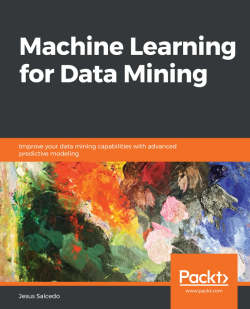### Machine Learning for Data Mining5 (1 reviews total)
By Jesus Salcedo

• Constantly updated with 100+ new titles each month
• Breadth and depth in over 1,000+ technologies

Machine learning (ML) combined with data mining can give you amazing results in your data mining work by empowering you with several ways to look at data. This book will help you improve your data mining techniques by using smart modeling techniques.

This book will teach you how to implement ML algorithms and techniques in your data mining work. It will enable you to pair the best algorithms with the right tools and processes. You will learn how to identify patterns and make predictions with minimal human intervention. You will build different types of ML models, such as the neural network, the Support Vector Machines (SVMs), and the Decision tree. You will see how all of these models works and what kind of data in the dataset they are suited for. You will learn how to combine the results of different models in order to improve accuracy. Topics such as removing noise and handling errors will give you an added edge in model building and optimization.

By the end of this book, you will be able to build predictive models and extract information of interest from the dataset

Publication date:
April 2019
Publisher
Packt
Pages
252
ISBN
9781838828974

# Introducing Machine Learning Predictive Models

A large percentage of data mining opportunities involve machine learning, and these opportunities often come with greater financial rewards. This chapter will give you the basic knowledge that you need to bring the power of machine learning into your data mining work. In this chapter, we're going to talk about the characteristics of machine learning models and also see some examples of these models.

The following are the topics that we will be covering in this chapter:

• Characteristics of machine learning predictive models
• Types of machine learning predictive models
• Working with neural networks
• A sample neural network model

# Characteristics of machine learning predictive models

Knowing the characteristics of machine learning predictive models will help you understand the advantages and limitations in comparison to any statistical or decision tree models.

Let's get some insights on a few characteristics of predictive models in machine learning:

• Optimized to learn complex patterns: Machine learning models are designed to be optimized to learn complex patterns. In comparison to statistical models or decision tree models, predictive models greatly excel, when you have very complex patterns in data.
• Account for interactions and nonlinear relationships: Machine learning predictive models can account for interactions in the data and nonlinear relationships to an even better degree than decision tree models.
• Few assumptions: These models are powerful because they have very few assumptions. They can also be used with different types of data.
• A black box model's interpretation is not straightforward: Predictive models are black box models, this is one of the drawbacks of predictive machine learning models, because this implies that the interpretation is not straightforward. This means that, if we end up building many different equations and combine them, it becomes very difficult to see exactly how each one of these variables ended up interacting and impacting an output variable. So, the predictive machine learning models are great when it comes to predictive accuracy, but they're not that good for understanding the mechanics behind a prediction.

If you want to predict something, these models do a pretty good job and have amazing accuracy. But if you want to know why something is being predicted, and if you are looking forward to making some changes in the implementation so that you don't get a particular prediction, then it would be difficult to decipher.

# Types of machine learning predictive models

The following are some of the different types of machine learning predictive models:

• Neural networks
• Support Vector Machines
• Random forest
• Naive Bayesian algorithms
• K-nearest neighbors
• Self-learning response model

We won't be covering all of them, but we'll focus on a very interesting model – the neural network. In the following sections, we will get an in-depth view of what neural networks are.

# Working with neural networks

Neural networks were initially developed in an attempt to understand how the brain operates. They were originally used in the areas of neuroscience and linguistics.

In these fields, researchers noticed that something happened in the environment (input), the individual processed the information (in the brain), and then reacted in some way (output).

So, the idea behind neural networks or neural nets is that they will serve as a brain, which is like a black box. We then have to try to figure out what is going on so that the findings can be applied.

The following are the advantages of using a neural network:

• Good for many types of problems: They work well with most of the complex problems that you might come across.
• They generalize very well: Accurate generalization is a very important feature.
• They are very common: Neural networks have become very common in today's world, and they are readily accepted and implemented for real-world problems.
• A lot is known about them: Owing to the popularity that neural networks have gained, there is a lot of research being done and implemented successfully in different areas, so there is a lot of information available on neural networks.
• Works well with non-clustered data: When you have non-clustered data, neural networks can be used in several situations, such as where the data itself is very complex, where you have many interactions, or where you have nonlinear relationships; neural networks are certainly very powerful and very robust solutions for such situations.

Good models come at the cost of a few disadvantages:

• They take time to train: Neural networks do take a long time to train; they are generally slower than a linear regression model or a decision tree model, as these basically just do one pass on the data, while, with neural networks, you actually go through many, many iterations.
• The best solution is not guaranteed: You're not guaranteed to find the best solution. This also means that, in addition to running a single neural network through many iterations, you'll also need to run it multiple times using different starting points so that you can try to get closer to the best solution.
• Black boxes: As we discussed earlier, it is hard to decipher what gave a certain output and how.

# Representing the errors

While building our neural network, our actual goal is to build the best possible solution, and not to get stuck with a sub-optimal one. We'll need to run a neural network multiple times.

Consider this error graph as an example: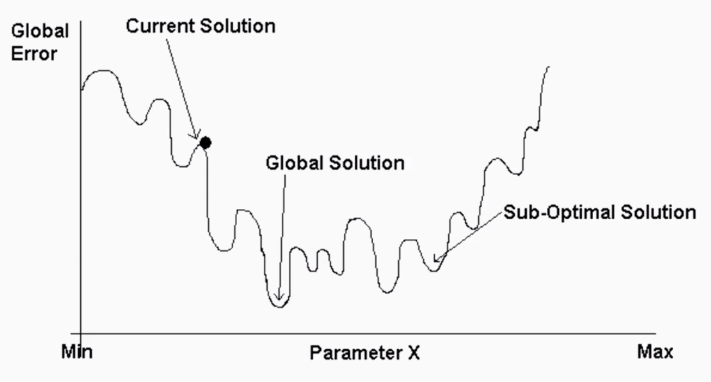This is a graph depicting the amount of errors in different solutions. The Global Solution is the best possible solution and is really optimal. A Sub-Optimal Solution is a solution that terminates, gets stuck, and no longer improves, but it isn't really the best solution.

# Types of neural network models

There are different types of neural networks available for us; in this section, we will gain insights into these.

# Multi-layer perceptron

The most common type is called the multi-layer perceptron model. This neural network model consists of neurons represented by circles, as shown in the following diagram. These neurons are organized into layers: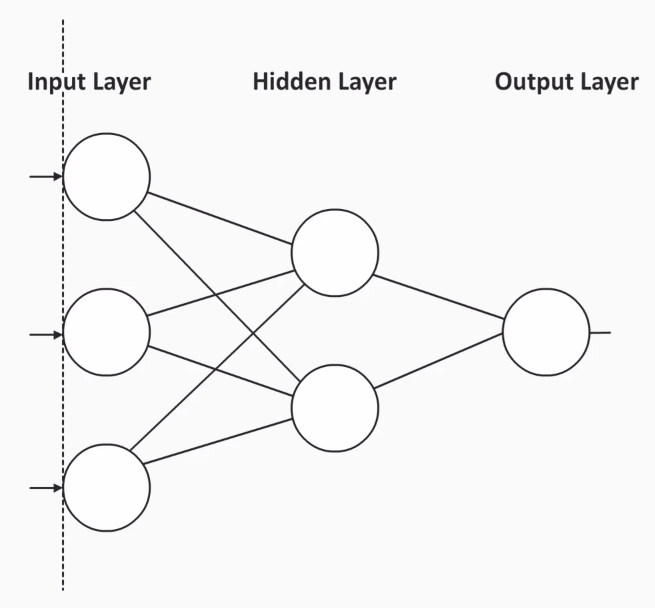Every multi-layer perceptron model will have at least three layers:

• Input Layer: This layer consists of all the predictors in our data.
• Output Layer: This will consist of the outcome variable, which is also known as the dependent variable or target variable.
• Hidden Layer: This layer is where you maximize the power of a neural network. Non-linear relationships can also be created in this layer, and all the complex interactions are carried out here. You can have many such hidden layers.

You will also notice in the preceding diagram that every neuron in a layer is connected to every neuron in the next layer. This forms connections, and every connecting line will have a weight associated with it. These weights will form different equations in the model.

# Why are weights important?

Weights are important for several reasons. First because all neurons in one layer are connected to every neuron in the next layer, this means that the layers are connected. It also means that a neural network model, unlike many other models, doesn't drop any predictors. So for example, you may start off with 20 predictors, and these 20 predictors will be kept. A second reason why weights are important is that they provide information on the impact or importance of each predictor to the prediction. As will be shown later, these weights start off randomly, however through multiple iterations, the weights are modified so as to provide meaningful information.

# An example representation of a multilayer perceptron model

Here, we will look at an example of a multilayer perceptron model. We will try to predict a potential buyer of a particular item based on an individual's age, income, and gender.

Consider the following, for example: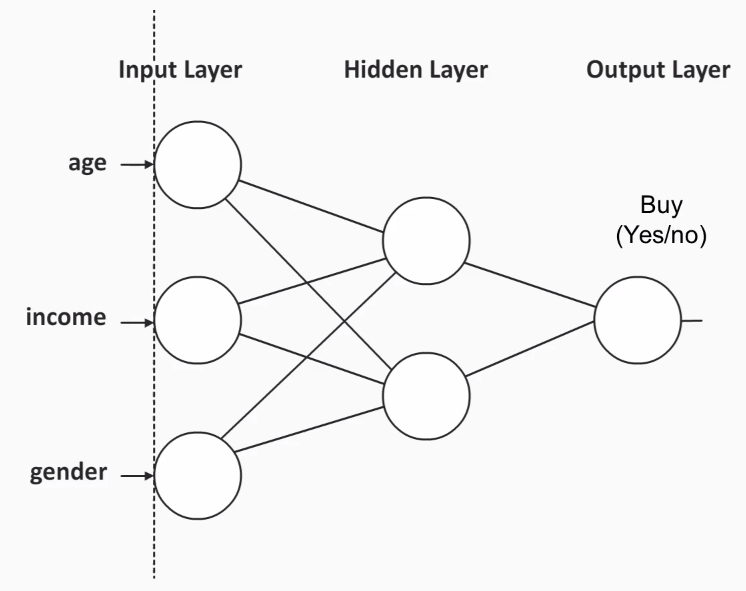As you can see, our input predictors that form the Input Layer are age, income, and gender. The outcome variable that forms our Output Layer is Buy, which will determine whether someone bought a product or not. There is a hidden layer where the input predictors end up combining.

To better understand what goes on behind the scenes of a neural network model, lets take a look at a linear regression model.

# The linear regression model

Let's understand the linear regression model with the help of an example.

Consider the following: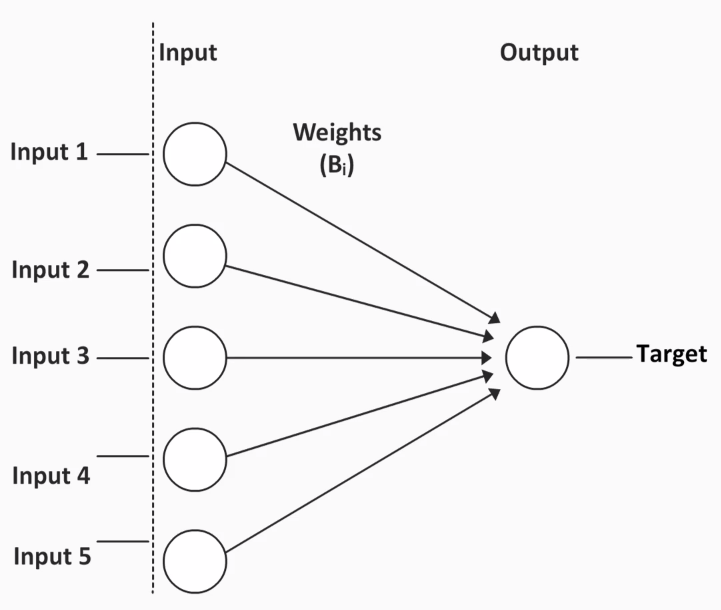In linear regression, every input predictor in the Input Layer is connected to the outcome field by a single connection weight, also known as the coefficient, and these coefficients are estimated by a single pass through the data. The number of coefficients will be equal to the number of predictors. This means that every predictor will have a coefficient associated with it.

Every input predictor is directly connected to the Target with a particular coefficient as its weight. So, we can easily see the impact of a one unit change in the input predictor on the outcome variable or the Target. These kind of connections make it easy to determine the effect of each predictor on the Target variable as well as on the equation.

# A sample neural network model

Let's use an example to understand neural networks in more detail: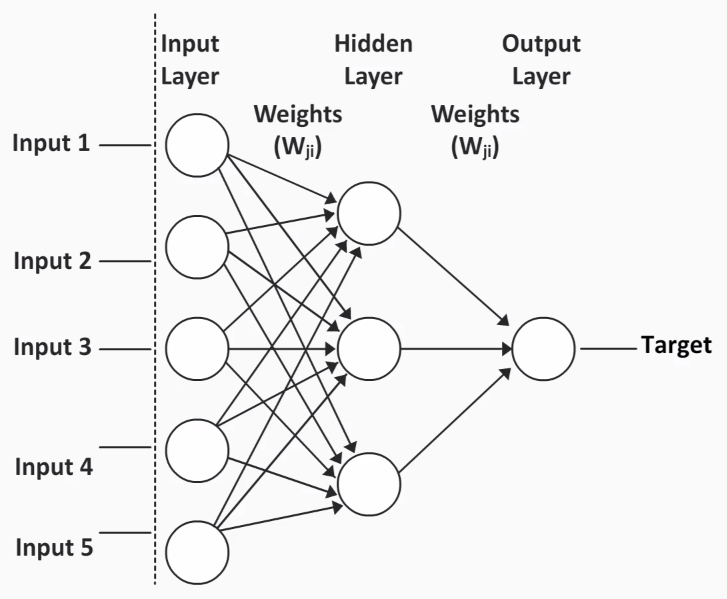Notice that every neuron in the Input Layer is connected to every neuron in the Hidden Layer, for example, Input 1 is connected to the first, second, and even the third neuron in the Hidden Layer. This implies that there will be three different weights, and these weights will be a part of three different equations.

This is what happens in this example:

• The Hidden Layer intervenes between the Input Layer and the Output Layer.
• The Hidden Layer allows for more complex models with nonlinear relationships.
• There are many equations, so the influence of a single predictor on the outcome variable occurs through a variety of paths.
• The interpretation of weights won't be straightforward.
• Weights correspond to the variable importance; they will initially be random, and then they will go through a bunch of different iterations and will be changed based on the feedback of the iterations. They will then have their real meaning of being associated with variable importance.

So, let's go ahead and see how these weights are determined and how we can form a functional neural network.

# Feed-forward backpropagation

Feed-forward backpropagation is a method through which we can predict things such as weights, and ultimately the outcome of a neural network.

According to this method, the following iterations occur on predictions:

• If a prediction is correct, the weight associated with it is strengthened. Imagine the neural network saying, Hey, you know what, we used the weight of 0.75 for the first part of this equation for the first predictor and we got the correct prediction; that's probably a good starting point.
• Suppose the prediction is incorrect; the error is fed back or back propagated into the model so that the weights or weight coefficients are modified, as shown here: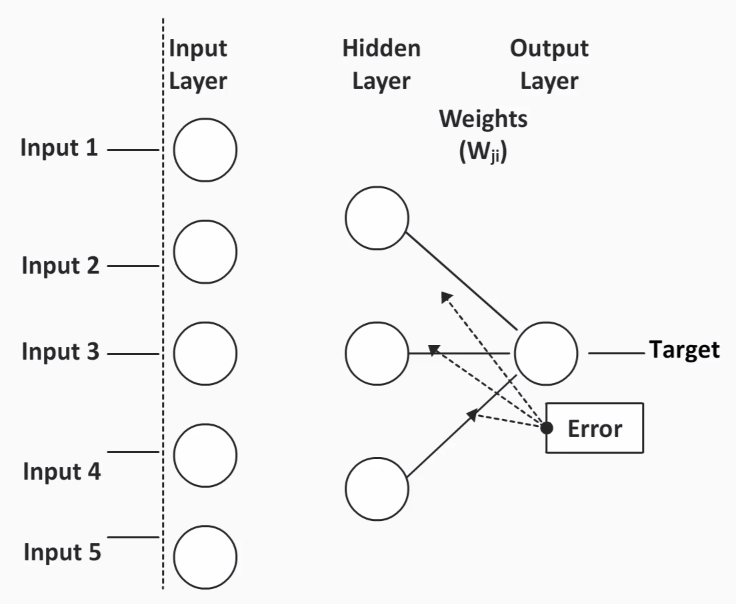This backpropagation won't just take place in-between the Hidden Layers and the Target layer, but will also take place toward the Input Layer: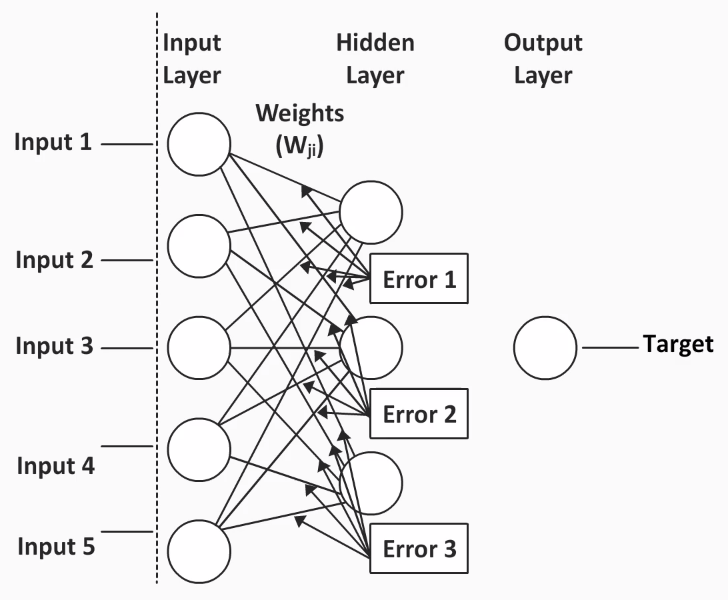While these iterations are happening, we are actually making our neural network better and better with every error propagation. The connections now make a neural network capable of learning different patterns in the data.

So, unlike any linear regression or a decision tree model, a neural network tries to learn patterns in the data. If it's given enough time to learn those patterns, the neural network, combined with its experience, understands and predicts better, improving the rate of accuracy to a great extent.

# Model training ethics

When you are training the neural network model, never train the model with the whole dataset. We need to hold back some data for testing purposes. This will allow us to test whether the neural network is able to apply what its learned from the training dataset to a new data.

We want the neural network to generalize well to new data and capture the generalities of different types of data, not just little nuances that would then make it sample-specific. Instead, we want the results to be translated to the new data as well. After the model has been trained, the new data can be predicted using the model's experience.

# Summary

I hope you are now clear on machine learning predictive models and have understood the basic concepts. In this chapter, we have seen the characteristics of machine learning predictive models and have learned about some of the different types. These concepts are stepping stones to further chapters. We have also looked at an example of a basic neural network model. In the next chapter, we will implement a live neural network on a dataset and you will also be introduced to support vector machines and their implementation.